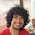Divisible by 289?

For how many integers x, x^2 - 3x - 19 is divisible by 289?

Perfect square?

For how many positive integral values of n, the expression n(4n + 1) is a perfect square?

Update (23/01/10)(05/02/10): Solution: Posted by Aaditya Ramdas (CSE IITB Alumnus & Tower Research), Piyush Sao (Senior Undergrad, EE, IITM) and Nikhil Garg (Sophomore, IITD) in comments!!  Thanx.

1.f(x)=x^2-3^x-19
=x^2-3x-70 +51
= ( x-10) (x+7 ) + 51

Now as f(x) is divisible by 289 => it is divisile by 17.
=> (x-10) (x+7) is divisible by 17
=> either (x-10) is divisible by 17 or/and ( x+7) is divisible by 17

Even if one of them is divisible by 17 , other is too. ( 10 +7 =0(mod 17) )

=> (x-10)(x+7) is divisble by 17*17

=> f(x) is always 51(mod 289 )

=> no solution

2.FIRST one no solution: my solution in a way isomorphic to above solution.

n(4n+1) can't be perfect square as gcd(n,4n+1)=1 hence both should be sqaure so if n=k^2 then trivially 4k^2+1 can't be square.

3.Nice solutions.. Thanx

I was not expecting quick answers. First one was really tough. For the second, I didn't have elegant solution like you do.

For the second question:
Let n(4n+1) = k^2; (We can see that k>2n)
k^2 - 4n^2 = n;
(k-2n)(k+2n) = n;
The difference between two positive factors is 4n, which is not possible as the factors of n are all less than n. :)

Hence proved that no perfect square. :)

But sure, Piyush's solution is far better than mine.

4.i didnt get the first one. the second one was pretty trivial - just note this - the expression u gave was 4n^2 + n. But (2n)^2 is 4n^2 and (2n+1)^2 is 4n^2 + 4n +1. So obviously there is no perfect square 4n^2 + n. chamka? :P

5.@Ramdas.. of course.. that was "too" simple.. I could not think in this direction.. instead did it the way piyush sao did :P

For the first part, reposting Nikhil's solution:
Find values of integers x such that x^2 - 3x-19 is divisible by 289. We will prove that no such x exists.

x^2 - 3*x - 19 = (x-10)(x+7) + 51

For (x-10)(x+7) + 51 to be divisible by 289, (x-10)(x+7) has to be divisible by 17.

So, atleast one of (x-10) or (x+7) is divisible by 17.

Note that (x+7) - (x-10) = 17. So, if at all there exists an x, at that x, both are divisible by 17.

So, the function is 17*17*(...) + 51. So, the function x^2-3x-19 is of the form 289*(..) + 51
So, for no x, x^2-3x-19 is divisible by 289 :)

6.I did the first one with the use of quadratic equations and the answer is zero.

The second one has a simpler solution in :-
For any number n, the minimum difference between n^2 and the next square is 2*n - 1. In this case, n(4n+1) can be written as 4n^2 + n => (2n)^2 + n. The minimum difference between the next square in this case would be 2* (2n) - 1 i.e. 4n - 1. And since 4n-1 is always greater than n, there isn't a solution.